# IIT JAM Chemistry - MCQ Test 1

## 60 Questions MCQ Test MOCK Test Series for IIT JAM Chemistry | IIT JAM Chemistry - MCQ Test 1

Description
This mock test of IIT JAM Chemistry - MCQ Test 1 for Chemistry helps you for every Chemistry entrance exam. This contains 60 Multiple Choice Questions for Chemistry IIT JAM Chemistry - MCQ Test 1 (mcq) to study with solutions a complete question bank. The solved questions answers in this IIT JAM Chemistry - MCQ Test 1 quiz give you a good mix of easy questions and tough questions. Chemistry students definitely take this IIT JAM Chemistry - MCQ Test 1 exercise for a better result in the exam. You can find other IIT JAM Chemistry - MCQ Test 1 extra questions, long questions & short questions for Chemistry on EduRev as well by searching above.
*Multiple options can be correct
QUESTION: 1

### The most stable canonical structure among all of above is: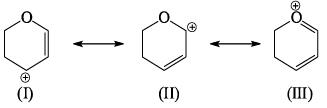Solution:

The structure which has maximum double bond is more stable.

QUESTION: 2

### Strongest base among the following species is:

Solution:

Nitrogen atom is more basic than other two oxygen and carbon . Also it has an available lone pair.

*Multiple options can be correct
QUESTION: 3

### Compare relative stability of the following structures: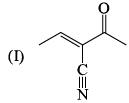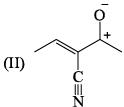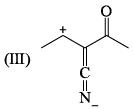Solution:

Structure 1 is uncharged and most stable.
Structure 2 has a charge separation and the two charges are on adjacent atoms.
Structure 3 is the least stable as its charges are separated over a large distance.

QUESTION: 4

Strength of following bases decrease in the order?

(I) Br   (II) F-      (III) NH2    (IV) CH3-

Solution:

br- 2.96
F- 3.98
NH2, The electronegativity of Nitrogen is 3.04 and Hydrogen is 2.2 So this difference makes the N-H bond polar.
CH3 = 5.25

QUESTION: 5

The ratio of van der Waal’s constant a and b,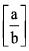has the dimensions of:

Solution:

The ratio of van der Waals’ constants a and b has the dimensions of  atm L mole-1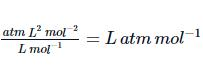Hence B is the correct answer

QUESTION: 6

Find the corresponding subshell utilizing the information from graph.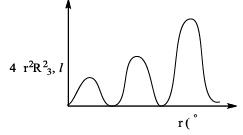Solution:

Radial Nodes = n - l – 1

= 3 – 0 – 1

=2 nodes. Therefore 3s

Hence A is the correct answer.

QUESTION: 7

Distribution of molecules with velocity is represented by curve as shown: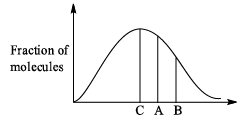Velocity at point A is:

Solution:
QUESTION: 8

Which have lowest bond angle?

Solution:
1.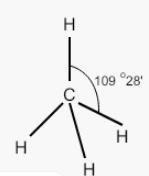2.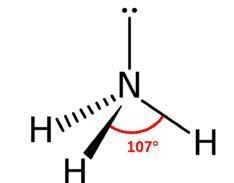3.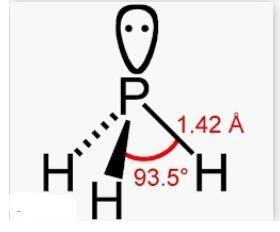4.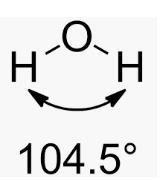5. PH3 because it follows Drago’s rule and hence there would be no or very low hybridization and hence pure p-orbital will be used. Therefore bond angle will be almost equal to 90 degree.

Hence C is correct.

QUESTION: 9

Of the following acids, the one that is strongest is:

Solution:

Higher the oxidation no. of the central atom, stronger is the acid.
Here, Br=+7
Cl=+1
N=+3
P=+3
Thus HBrO4 is the strongest acid amongst the given alternatives

*Multiple options can be correct
QUESTION: 10

Lattice energy (numerical value) of chloride of alkali metals is in order:

Solution:

Lattice energy is the attraction force acting between the cation and anion.

Greater the size of cation, lower is the attractive force.

On moving down the group, size of cation increases then,

1.  Attractive force decreases.
2.  Lattice energy decreases.
*Multiple options can be correct
QUESTION: 11

Which of the following drawings is not a resonance structure of 1 -nitrocyclohexene: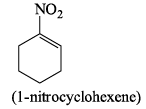Solution:
QUESTION: 12

Which of the following is not resonating structure of each other?

Solution:

Molecules in option (a) are linkage isomers of each other.

According to rules of Resonance, no change in position of atom should take place.

QUESTION: 13

Examine the following resonating structures of formic acid for their individual stability and then answer the question given below: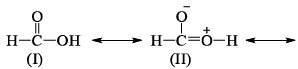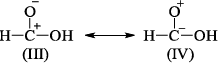Which of the following arrangements gives the correct order of decreasing stability of the above- mentioned resonance contributors?

Solution:

There are certain sets of rules that must be followed in order to check the stability of resonating structures.

These rules have a preference so we have to follow the order

1. Uncharged species are more stable than charged species.

2. Charged species having more number of covalent bond are more stable.

3. The species in which octet rule is followed by every atom is more stable than the species in which octet rule is violated.

4. Species in which opposite charges are closer are more stable than species having same charges closer.

5. Species with negative charge on more electronegative atoms while positive charge on more electropositive atoms are more stable.

According to the above discussion, B is the correct answer.

QUESTION: 14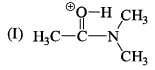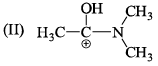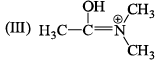The correct stability order of the given resonating structures is:

Solution:

Explanation : Valence shell = 6e-

In II, octet is incomplete

E.N : O > N

The correct sequence is : III > I > II

QUESTION: 15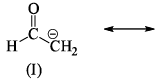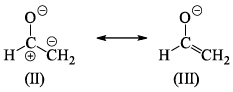The correct order of stability for the given canonical structures is:

Solution:

There are certain set of rules that must be followed in order to check the stability of resonating structures.

These rules have a preference so we have to follow the order

1. Uncharged species is more stable than charged species.

2. Charged species having more number of covalent bond are more stable.

3. The species in which octet rule is followed by every atom is more stable than the species in which octet rule is violated.

4. Species in which opposite charges are closer are more stable than species having same charges closer.

5. Species with negative charge on more electronegative atom while positive charge on more electropositive atom is more stable.

According to above discussion, B is the correct answer.

QUESTION: 16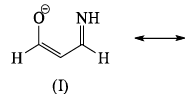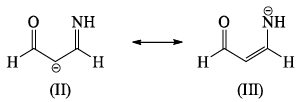The correct order of stability among the following canonical structure is:

Solution:

There are certain set of rules that must be followed in order to check the stability of resonating structures.

These rules have a preference so we have to follow the order

1. Uncharged species is more stable than charged species.

2. Charged species having more number of covalent bond are more stable.

3. The species in which octet rule is followed by every atom is more stable than the species in which octet rule is violated.

4. Species in which opposite charges are closer are more stable than species having same charges closer.

5. Species with negative charge on more electronegative atom while positive charge on more electropositive atom is more stable.

According to above discussion, D is the correct answer.

QUESTION: 17

Which of the following is most basic?

Solution:

Basicity is the tendency of an atom to donate their lone pair.

In case of  (a), (b),(d), lone pair of Nitrogen atom is in conjugation which is not available to be donated.

Hence (c) is more basic.

*Multiple options can be correct
QUESTION: 18

If a particle in the box of length 'l' has wavelength, ψ = (l -x)x. (x is only variable)  What is its normalization constant:

Solution:
QUESTION: 19

Match column I with column II and select the correct answers: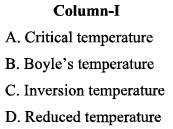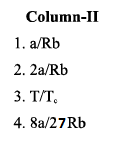Solution:
1. A critical point (or critical state) is the end point of a phase equilibrium curve.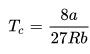2. The Boyle temperature is the temperature at which a non ideal gas behaves most like an ideal gas.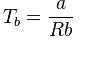3. The characteristic temperature below which a gas expands adiabatically into a region of low pressure through a porous plug with a fall in temperature is called as inversion temperature (Ti).  Ti =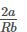4. The reduced temperature of a fluid is its actual temperature, divided by its critical temperature.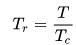*Multiple options can be correct
QUESTION: 20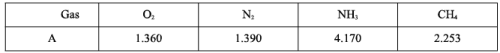The table indicates the value of van-der Waal’s constant ‘a’. The gas which can be liquefied most easily is:

Solution:

The ease of liquification of a gas depends on their intermolecular force of attraction which in turn is measured in terms of van der Waals’ constant ‘a’. Hence, higher the value of ‘a’, greater the intermolecular force of attraction, easier the liquification. In the present case, NH3 has highest ‘a’, can most easily be liquefied.

*Multiple options can be correct
QUESTION: 21

rms velocity of hydrogen is √7 times the rms velocity of nitrogen. If T is the temperature of gas,then:

Solution: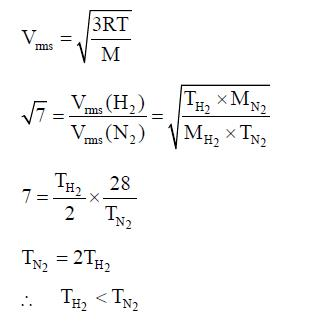*Multiple options can be correct
QUESTION: 22

Copper containing alloy weighing 0.3175g dissolved in an acid, and an excess of KI is added.
Estimation of copper in alloy is based on following reactions: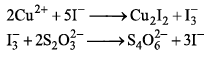Solution:

Iodometric determination of copper is based on the oxidation of iodides to iodine by copper (II) ions, which get reduced to Cu+.

Comparison of standard potentials for both half reactions (Cu2+/Cu+ E0=0.17 V,  I2/I- E0=0.54 V) suggests that it is iodine that should be acting as oxidizer. However, that's not the case, as copper (I) iodide CuI is very weakly soluble (Ksp = 10-12). That means concentration of Cu+ in the solution is very low and the standard potential of the half reaction Cu2+/Cu+ in the presence of iodides is much higher (around 0.88 V)

*Multiple options can be correct
QUESTION: 23

If a particle has linear momentum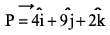at position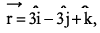, then its angular momentum is:

Solution:

Angular momentum is equal to the cross product of the position vector and the linear mamentum.calculate by vector cross product rule,you get the answer.

*Multiple options can be correct
QUESTION: 24

Consider two molecules A & B.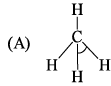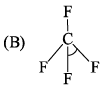∠α = ∠HCH; ∠β = ∠FCF

Which of following is true?

Solution:

Though the substituting atoms are different in both cases but the hybridization of carbon is the same that is sp3, having the bond angle of 109′28″.

QUESTION: 25

Predict the shape of IF4+ molecule using VSEPR theory:

Solution:

The electron geometry of IF4+ is trigonal bipyramidal. This is because it has five total electron groups. The central atom is bonded to four other atoms and has one lone pair of electrons. The lone pair of electrons will affect the molecular geometry of the compound as well as the bond angles.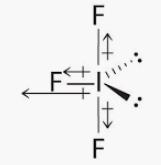*Multiple options can be correct
QUESTION: 26

Which of the following is true for ionization energy:

Solution:

The ionization energy of molecular nitrogen is 1503 kJ/mol, and that of atomic nitrogen is 1402 kJ/mol. We conclude that the energy of the electrons in molecular nitrogen is lower than that of the electrons in the separated atoms, so the molecule is bound.

*Multiple options can be correct
QUESTION: 27

XeO2F2 has shape:

Solution:

XeO2F2 molecular geometry is originally said to be trigonal bipyramidal but due to the presence of lone pair on equatorial position, the actual shape will be see-saw. The repulsion between bond pair and lone pair of electrons will be more.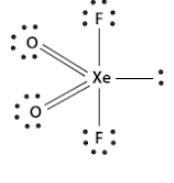*Multiple options can be correct
QUESTION: 28

What is the correct increasing order of bond lengths of bond indicated in following compund?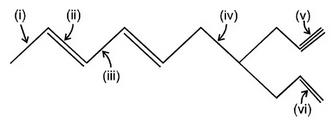Solution:
*Multiple options can be correct
QUESTION: 29

Arrange the following in decreasing order of their bond angles: NH3, H2O , CH4

Solution:

CH4 (109.28) > NH3(107) > H2O(104.5)

QUESTION: 30

The ground state electronic configuration of valence shell electrons in a molecule A2 is written as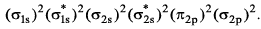• Hence bond order is_____________________.

Solution:

Bond Order =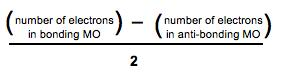Number of electrons in bonding MO = 8

Number of electrons in anti-bonding MO = 4

Bond order = (8 - 4)/2

Bond order = 4/2

Bond order = 2

*Multiple options can be correct
QUESTION: 31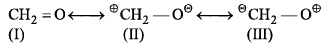Which of these structures is practically not a valid resonance structure for formaldehyde?

Solution: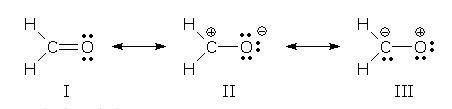The lowest energy form is I, because every atom has a complete octet. It is the major contributor.

II is lower in energy than III, because the negative charge is in the more electronegative O atom.

*Multiple options can be correct
QUESTION: 32

Which of the following is an electrophile?

Solution:

Electrophile is an electron pair acceptor. Electrophiles are positively charged or neutral species having vacant orbitals that are attracted to an electron rich centre. It participates in a chemical reaction by accepting an electron pair in order to bond to a nucleophile.

*Multiple options can be correct
QUESTION: 33

Select the correct statement:

Solution:

Benzoic acid is a stronger acid than phenol because the benzoate ion is stabilised by two equivalent resonance structures in which the negative charge is present at the more electronegative oxygen atom.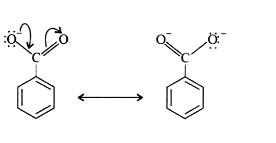The conjugate base of phenol, a phenoxide ion, has non-equivalent resonance structures in which the negative charge is at the less electronegative carbon atom. Thus, the benzoate ion is more stable than phenoxide ion. Hence, benzoic acid is a stronger acid than phenol.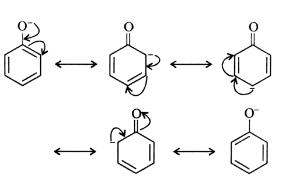*Multiple options can be correct
QUESTION: 34

An operator ‘A’ is defined as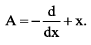Which one of the following statement is true?

Solution:
*Multiple options can be correct
QUESTION: 35

Identify which of the following operator is Hermitian:

Solution:
*Multiple options can be correct
QUESTION: 36

Which of the following is/are true about zero point energy?

Solution:

Zero-point energy (ZPE) is the lowest possible energy that a quantum mechanical system may have. Unlike in classical mechanics, quantum systems constantly fluctuate in their lowest energy state as described by the Heisenberg uncertainty principle.

*Multiple options can be correct
QUESTION: 37

Which of the following is/are true about Uncertainty principle:

Solution:

A) As uncertainity principle tells us about the minimum uncertain value of position for a certain value of momentum or vice versa. Hence it doesn't put an upper but a lower bound.

B) The equation,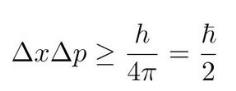is true under all conditions because it is the law of nature if anything exist it should have a certain amount of uncertainity relation, however it could be small in the bigger objects but it exists.

C) Both position and momentum are inversely proportional to each other .

D) If one of them become zero then the other should go infinity at the same time .

*Multiple options can be correct
QUESTION: 38

Which of the following is not a disproportionation reaction?

Solution:

A disproportionation reaction is when a multiatomic species whose pertinent element has a specific oxidation state gets oxidized and reduced in two separate half-reactions, yielding two other products containing the same pertinent element.

*Multiple options can be correct
QUESTION: 39

Consider the following cases:

(I) 60g CH3COOH (II) 3g HCHO (III) 60g NH2CONH2 (IV) 180g C6H12O6

Percentage of carbon is identical in:

Solution:

Percentage of an atom in any molecule can calculated by formula: n x (atomic weight/molecular weight) x 100

where n is total number of atomsin molecule

1) 60g CH3COOH

Atomic weight of carbon = 12

Molecular weight = 60

Number of atoms = 8

Percentage of atom =  n x (atomic weight/molecular weight) x 100

Percentage of atom = 8 x (12/60) x 100

Percentage of atom = 160

2) 3g HCHO

Atomic weight of carbon = 12

Molecular weight = 30

Number of atoms = 4

Percentage of atom =  n x (atomic weight/molecular weight) x 100

Percentage of atom = 4 x (12/30) x 100

Percentage of atom = 160

3) 60g NH2CONH2

Atomic weight of carbon = 12

Molecular weight = 62

Number of atoms = 8

Percentage of atom =  n x (atomic weight/molecular weight) x 100

Percentage of atom = 8 x (12/62) x 100

Percentage of atom = 154.2

4) 180g C6H12O6

Atomic weight of carbon = 12

Molecular weight = 180

Number of atoms = 24

Percentage of atom =  n x (atomic weight/molecular weight) x 100

Percentage of atom = 24 x (12/180) x 100

Percentage of atom = 160

*Multiple options can be correct
QUESTION: 40

In trigonal bipyramidal geometry, which of the following holds true?

Solution:

The axial bonds are longer than equatorial bonds because of greater repulsion from equatorial bonds. The 2-axial bonds are at 90º degree angle to 3-equatorial bonds while all equatorial bonds are at 120º angle to each other. So, none of the statements is correct hence option(d)

*Answer can only contain numeric values
QUESTION: 41

Total number of nucleophiles among following molecules is/are___________.
CH3O-, carbene, Nitrene, H2O , ROH, ZnCl2, NHR2, SnCl4-NH2 , Cl-

Solution:

Nucleophile is a chemical species that donates an electron pair to form a chemical bond in relation to a reaction. All molecules or ions with a free pair of electrons or at least one pi bond can act as nucleophiles. Because nucleophiles donate electrons, they are by definition Lewis bases

*Answer can only contain numeric values
QUESTION: 42

Total number of a-hydrogen in the given following compound is: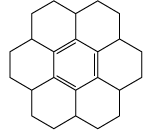Solution:
*Answer can only contain numeric values
QUESTION: 43

Number of carbenes among following:

:HgCl2, :CCl2 ,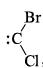, :CCl3, :SnCl2, BeCl2, :CH2.

Solution:

A carbene is a molecule containing a neutral carbon atom with a valence of two and two unshared valence electrons. The general formula is R-(C:)-R' or R=C: where the R represent substituents or hydrogen atoms.

*Answer can only contain numeric values
QUESTION: 44

A particle having energy 66h2/8mL2 for a 3D box has degeneracy?

Solution:
*Answer can only contain numeric values
QUESTION: 45

Find ΔE for states n = 3,4 in Bohr’s model of hydrogenic atoms (in eV):

Solution: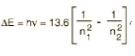ΔE = 13.6 [ 1/9 - 1/16]

ΔE = 13.6 [7/144]

ΔE = 13.6 x 0.048

ΔE = 0.66

*Answer can only contain numeric values
QUESTION: 46

By what factor does the average velocity of a gaseous molecule increase, when temperature is doubled:

Solution: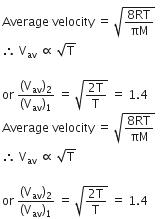*Answer can only contain numeric values
QUESTION: 47

What is the value of PV/nRT for ideal gas?

Solution:

Ideal gas equation, PV = nRT

Dividing both sides by nRT

⇒ PV/nRT = nRT/nRT

⇒ PV/nRT = 1

QUESTION: 48

Normality of a solution obtained by mixing 10 mL of  N/5 HCl & 30 mL of N/10 HCl is_______:

Solution: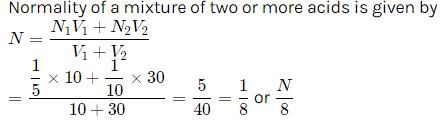*Answer can only contain numeric values
QUESTION: 49

Ca(OH)2 + H3PO4 → CaHPO4 + 2H2

Equivalent weight of H3PO4 in the above reaction is:

Solution:

H3PO4  is an acid and the equivalent mass of an acid is calculated by using the formula: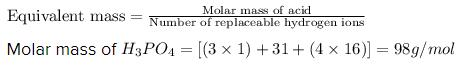From the given reaction:

Ca(OH)2 + H3PO4 → CaHPO4 + 2H2

As, the replaceable hydrogen ions in acid is 2, by putting the values in above equation, we get: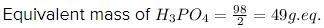Hence, The equivalent mass of H3PO4 for the given reaction is 49 g eq.

*Answer can only contain numeric values
QUESTION: 50

How many equivalents are there per mol of H2S in its oxidation to SO2?

Solution: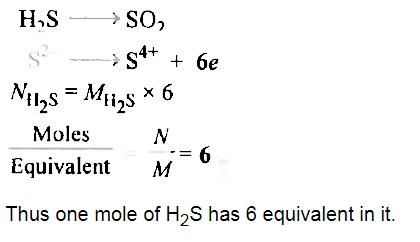*Answer can only contain numeric values
QUESTION: 51

Total number of the molecules that violates the rules of resonance are__________________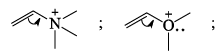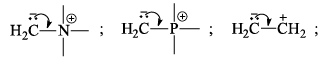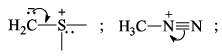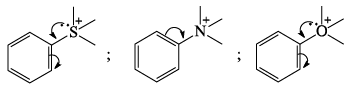Solution: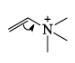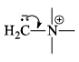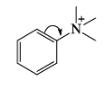In the above structure Nitrogen is having 5 bonds which is not possible, hence these molecules are violating the rules of resonance.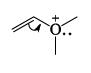In above molecule Oxygen is forming 4 bonds that is not possible, hence this molecules is also violating the rules of resonance.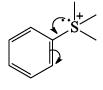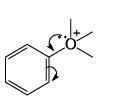In both molcules electron pair is moving from Sulphur and Oxygen which already have positive charge. Also in case of 2nd molecule, Oxygen is forming 5 bonds which is again not possible.

*Answer can only contain numeric values
QUESTION: 52

Total number of delocalized lone pairs (combined) in Adenine & Guanine: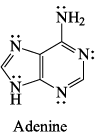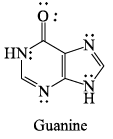Solution:
*Answer can only contain numeric values
QUESTION: 53

Number of electrophiles in following:

BF3, +CH3, -NH2, I+, PCl5, NH3, OH2, SnCl4, ROR

Solution:

In organic chemistry, an electrophile is an electron pair acceptor. Electrophiles are positively charged or neutral species having vacant orbitals that are attracted to an electron rich centre. It participates in a chemical reaction by accepting an electron pair in order to bond to a nucleophile. Because electrophiles accept electrons, they are Lewis acids. Most electrophiles are positively charged, have an atom that carries a partial positive charge, or have an atom that does not have an octet of electrons. They appear to attract electrons as well and seem to behave as though they are partially empty. These partially empty substances thus require an electron rich center, and thus they are filled. Electrophiles can be observed as electron-sensitive or photo-sensitive.

*Answer can only contain numeric values
QUESTION: 54

Find the probability of finding an e- in a box of length ‘L’ in middle half of box. (in %)

Solution:
*Answer can only contain numeric values
QUESTION: 55

At what temperature (in K), will the total KE of 0.30 mole of He be same as the total KE of 0.40 mole of Ar at 400 K?

Solution: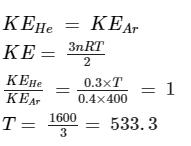*Answer can only contain numeric values
QUESTION: 56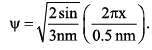What is the value of n2?

[Given is the wavefunction for a particle in 1-D box of length ‘L' If energy of ‘nth’ state is given by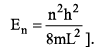Solution:
*Answer can only contain numeric values
QUESTION: 57

Number of molecules in 1L of water at 4°C is close to: (Give the multiple of NA)

Solution:

1 lit = 1000 cm3 = 1000 × 1 g = 1000 g = 1000/18 mol =55.5556 mol . It Contain 55.5556 N(A) number of molecule.

*Answer can only contain numeric values
QUESTION: 58

Consider AB2 molecule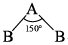Calculate % s-character in A—B bond:

Solution:

From Bent’s Rule equation,  cosx = s/s-1

where x is an bond angle and s is the % s character in the bond, it is clear that if we increase bond angle, the %s Character will be maximum.

Here angle is 150,

⇒ Cosx = s/(s-1)

⇒ Cos 150° = s/(s-1)

⇒ -0.86 = s/(s-1)

⇒ -0.86s +0.86 = s

⇒ 0.86 = 1.86s

⇒ s = 0.86/1.86

⇒ s = 0.462

⇒ %s = 0.462 X 10

⇒ %s = 46.2%

*Answer can only contain numeric values
QUESTION: 59

Number of π-bonds in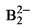will be:

Solution:
*Answer can only contain numeric values
QUESTION: 60

Concentration of 10% CH3COOH (w/v) in mol L-1 will be ________?

Solution:

M.W of acetic acid is 60 gm

10% (w/v) acetic acid means.
In 100 ml solution acetic acid is = 10 gm
so, in 100 ml solution acetic acid is = 10/60 mole.
so. in 1000ml solution acetic acid is = (0.167 × 1000)/100
= 1.67 mole / lit